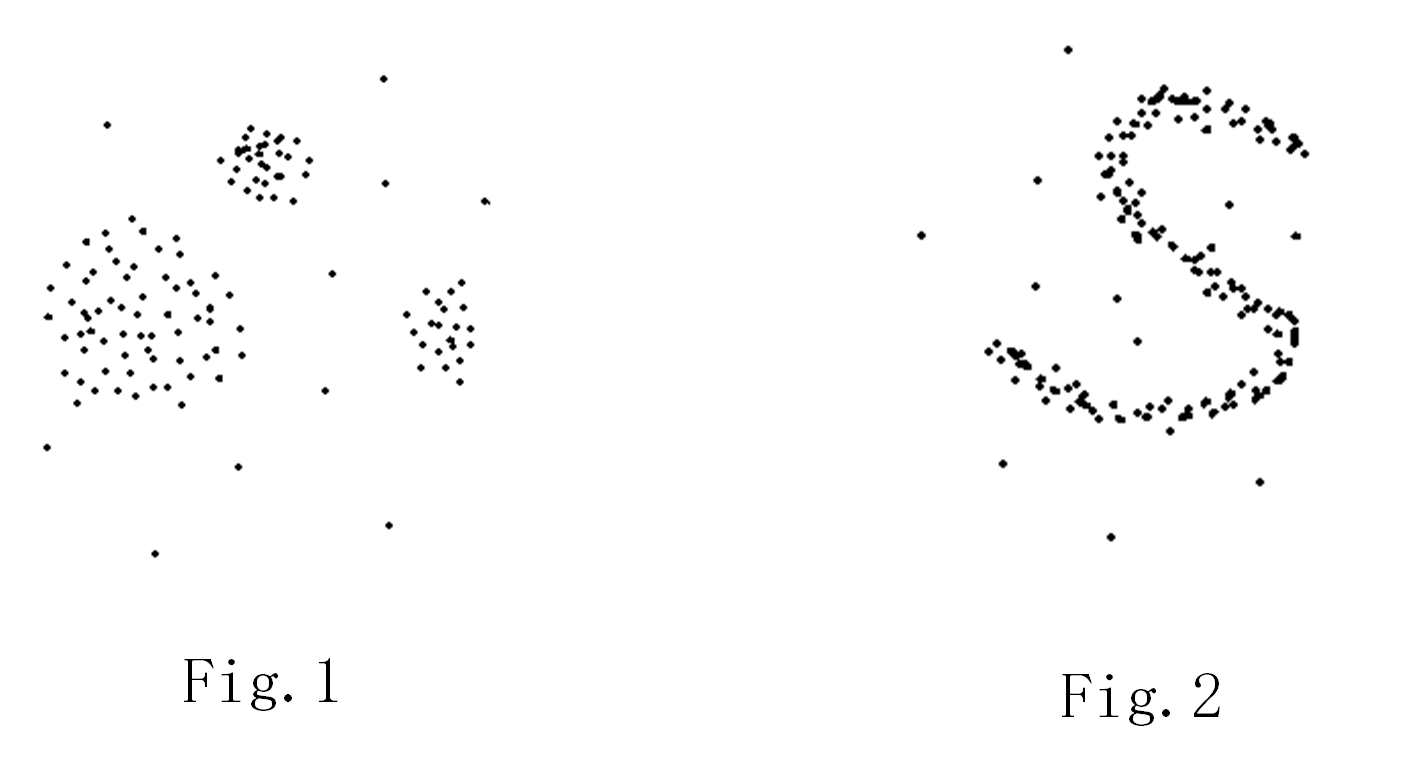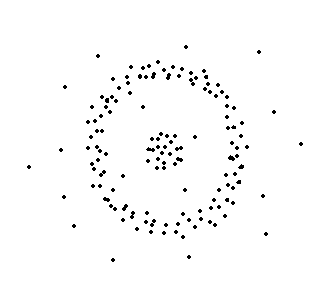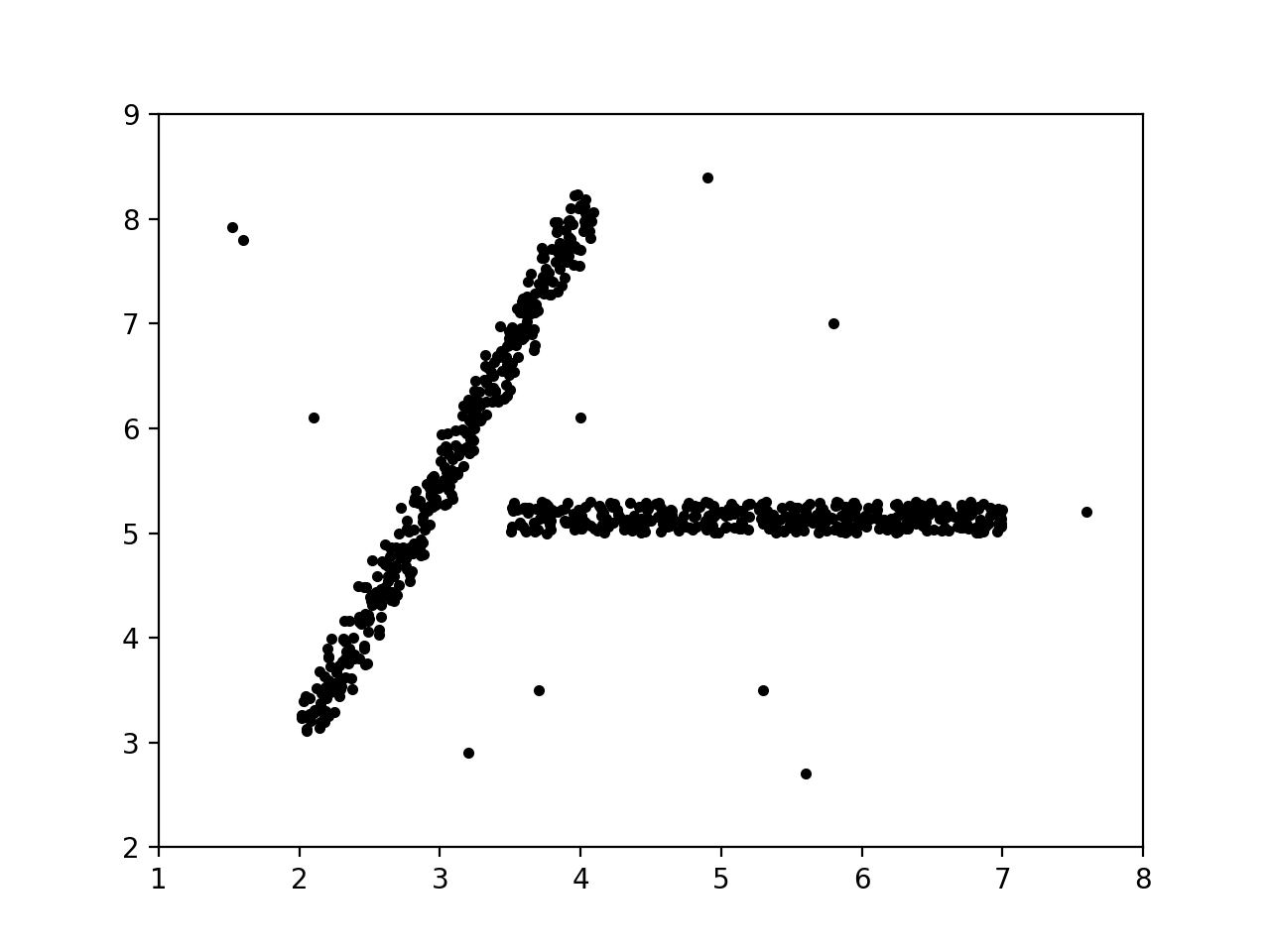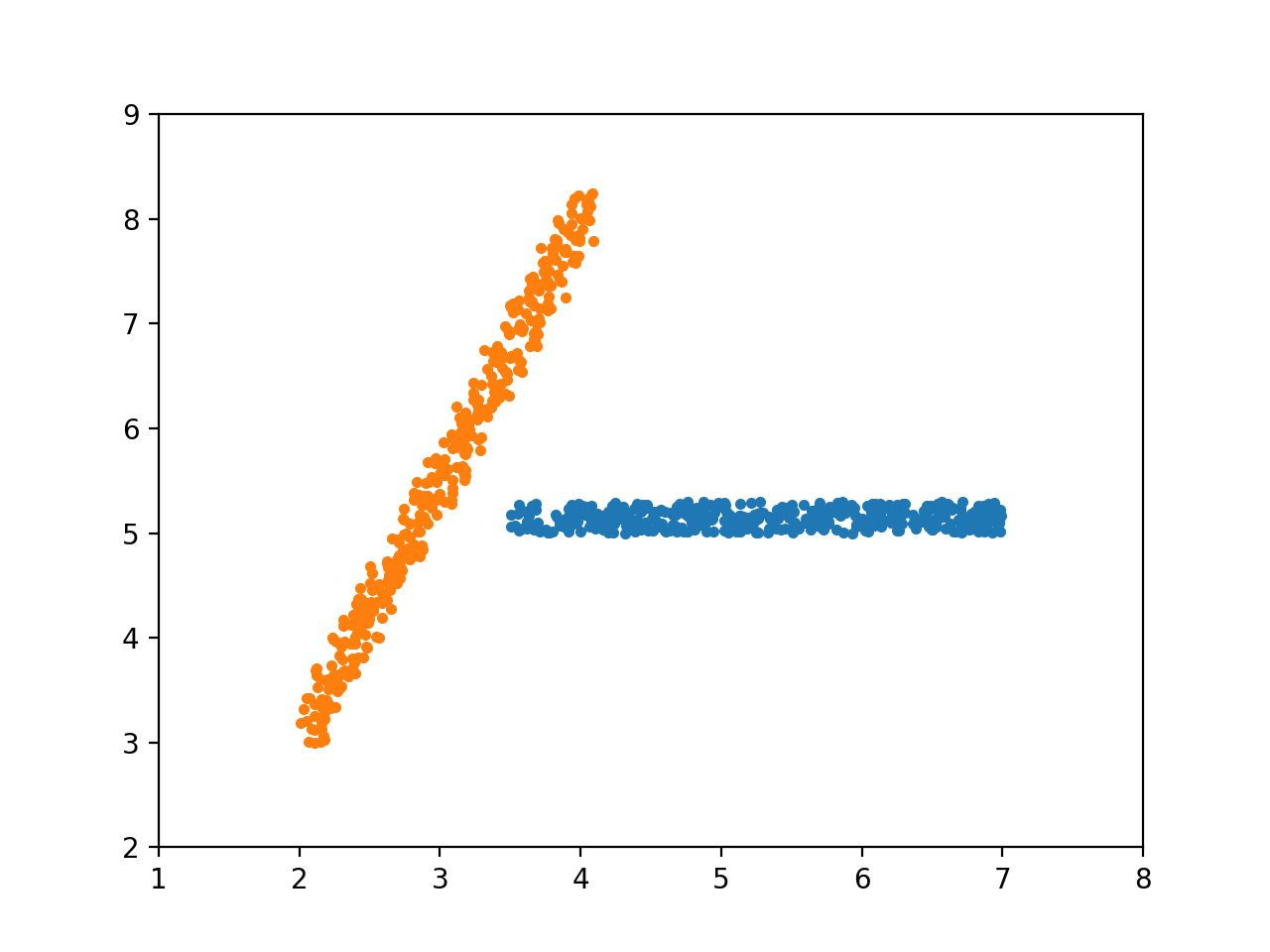# 聚类分析：基于密度聚类的DBSCAN算法机器学习 同时被 3 个专栏收录18 篇文章 5 订阅## 概念介绍

• 核心对象：一个数据点可被称为核心对象，如果以这个数据点为球心，以长度 ϵ ϵ $\epsilon$为半径的超球面区域内，至少有 MinPts M i n P t s $MinPts$个数据点。为了后面叙述简单，我将这个核心对象称为 (ϵ,MinPts) ( ϵ , M i n P t s ) $(\epsilon, MinPts)$-核心对象，将它的这个球面区域称为核心对象的 ϵ ϵ $\epsilon$-邻域。

• 直接密度可达：对于 (ϵ,MinPts) ( ϵ , M i n P t s ) $(\epsilon, MinPts)$-核心对象 O1 O 1 $O_1$和对象 O2 O 2 $O_2$，我们说 O2 O 2 $O_2$是从 O1 O 1 $O_1$直接密度可达的，如果 O2 O 2 $O_2$ O1 O 1 $O_1$ ϵ ϵ $\epsilon$-邻域内。注意，这里只要求 O1 O 1 $O_1$是核心对象，而 O2 O 2 $O_2$可以是任意对象（数据点）。也就是说，定义“A从B（或从B到A）直接密度可达”中，作为出发点的 B B $B$一定得是核心对象。

• 密度可达：从核心对象${O}_{1}$$O_1$到对象 On O n $O_n$（这里同样不要求 On O n $O_n$是核心对象）是密度可达的，如果存在一组对象链 {O1,O2,,On} { O 1 , O 2 , … , O n } $\{O_1, O_2, \dots, O_n\}$，其中 Oi O i $O_i$ Oi+1 O i + 1 $O_{i + 1}$都是关于 (ϵ,MinPts) ( ϵ , M i n P t s ) $(\epsilon, MinPts)$直接密度可达的。实际上，从这个定义我们不难看出， {O1,O2,,On1} { O 1 , O 2 , … , O n − 1 } $\{O_1, O_2, \dots, O_{n - 1}\}$都必须是核心对象。

• 密度相连：两个对象 O1,O2 O 1 , O 2 $O_1, O_2$（注意，这里不要求是核心对象）是密度相连的，如果存在一个对象 p p $p$，使得$p$$p$ O1 O 1 $O_1$ p p $p$${O}_{2}$$O_2$都是密度可达的。这个定义是对称的，即 O1 O 1 $O_1$ O2 O 2 $O_2$是密度相连的，则 O2 O 2 $O_2$ O1 O 1 $O_1$也是密度相连的。

• 噪声对象：不是核心对象，且也不在任何一个核心对象的 ϵ ϵ $\epsilon$-邻域内；
• 边缘对象：顾名思义是类的边缘点，它不是核心对象，但在某一个核心对象的 ϵ ϵ $\epsilon$-邻域内；

## 算法思路1.在所有属性为unvisited的点集中，随机找一个点 p p $p$，标记为visited

2.检查它是否为核心对象，若不是，标记$p$$p$为噪声点，再重新开始寻找（像第1步中那样）；若是，则执行后面的步骤；

3.建立一个候选集$Candidates$$Candidates$，初始时， Candidates C a n d i d a t e s $Candidates$中只有一个元素，那就是上一步找到的核心对象；

4.对于 Candidates C a n d i d a t e s $Candidates$中的每一个对象（记为 p p $p$），做以下操作：
(1) 若$p$$p$还未被聚到任意一个类，则加入类 C C $C$
(2) 若$p$$p$是核心对象，则将它的 ϵ ϵ $\epsilon$-邻域内的，除了当前类 C C $C$的点之外的点（也就是$Candidates-C$$Candidates - C$）加入 Candidates C a n d i d a t e s $Candidates$
(3) 若 p p $p$在噪声集合内，将它从噪声集合移除（因为肯定不是噪声）；
(4) 若$p$$p$的属性是unvisited，则标记为visited

5.循环执行第4步，直到 Candidates C a n d i d a t e s $Candidates$中找不到核心对象为止，这说明当前这个类已经被找完全了。

6.返回第1步，开始找下一个簇。直到所有的点都变成visited.

## 代码实现import numpy as np

class DataPoint(object):
def __init__(self, coordinates):
"""
:param coordinates: the ndarray object
"""
self.coordinates = coordinates
self.neighborhood = set()

"""

:param db: the database whose elements are DataPoint objects.
:param minPts:
:return:
"""
for i in db:
if np.linalg.norm(self.coordinates - i.coordinates) <= radius:

if len(self.neighborhood) >= minPts:
return True
return False


def dbscan(db, radius, minPts):
"""

:param db:
:param minPts:
:return:
"""
visited = set()
noise = set()
results = []

while len(visited) < len(db):

core = None
C = set()

# find the core point
temp = set()
for point in db - visited:
if point.isCore(db - {point}, radius, minPts):
core = point
break
else:
visited.update(temp)

# can't find any core point, i.e., the cluster process should be terminated
if not core:
break

# construct the new cluster C
flag = True
candidates = {core}

# flag denotes whether there is at least one core point in candidates
while flag:

flag = False
tempCandidates = set()
for member in candidates:

# remove the boundary points in noise
if member in noise:
noise.remove(member)

# label the cur as visited
if member not in visited:

# if cur not belongs to other clusters, add it in the cluster C
belongToOtherCluster = False
for cluster in results:
if member in cluster:
belongToOtherCluster = True
break
if not belongToOtherCluster:

# if cur is core, add its neighborhood in tempCandidates
if member.isCore(db - {member}, radius, minPts):
tempCandidates.update(member.neighborhood - C)
flag = True
candidates = set() | tempCandidates
results.append(C)
return results09-102万+12-22
12-276588
05-06180
06-261万+
06-284811
07-112万+
01-294829
08-21317
02-251万+
12-04473
09-111854
09-203万+
03-0127万+
06-20613点击重新获取扫码支付余额充值